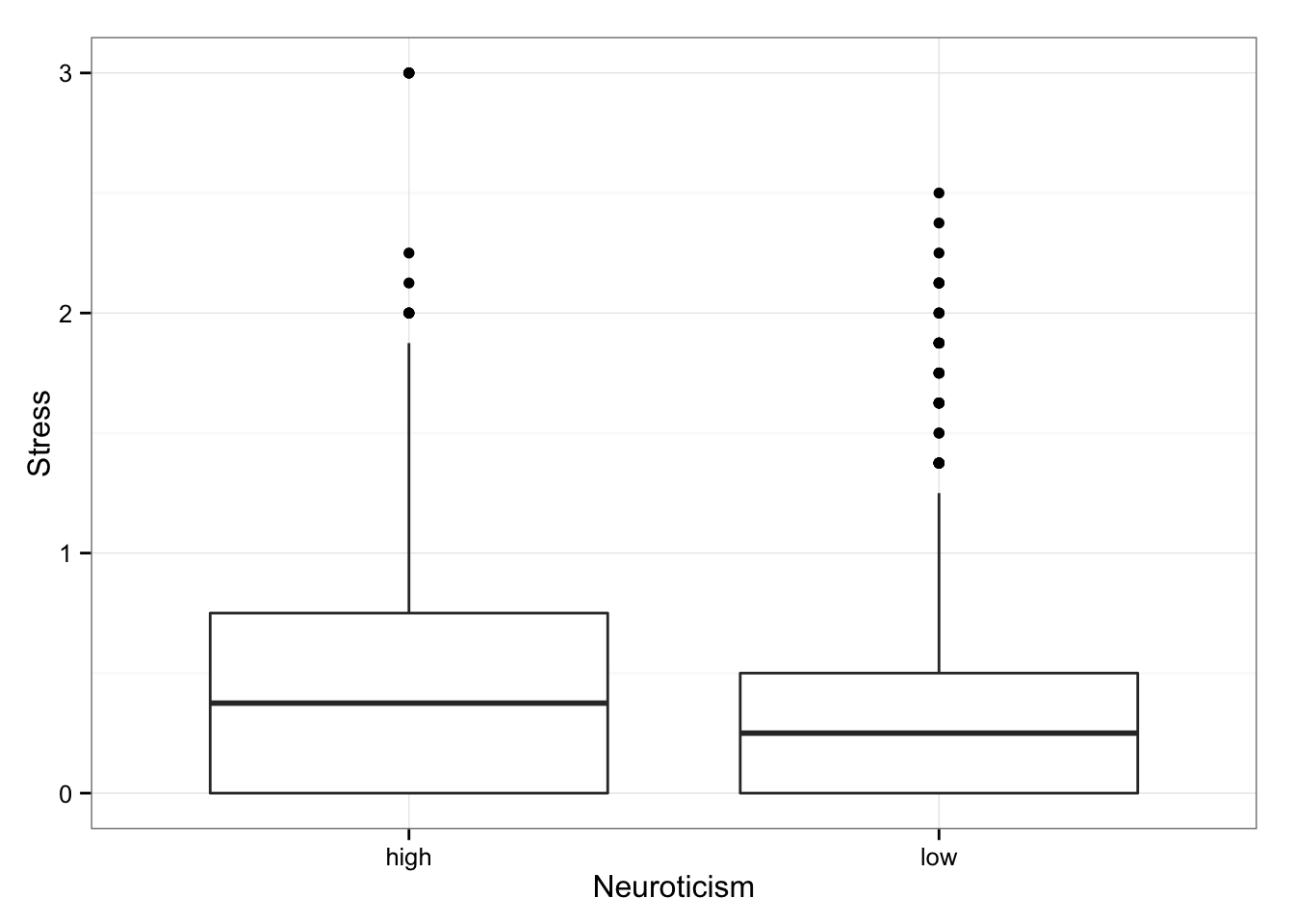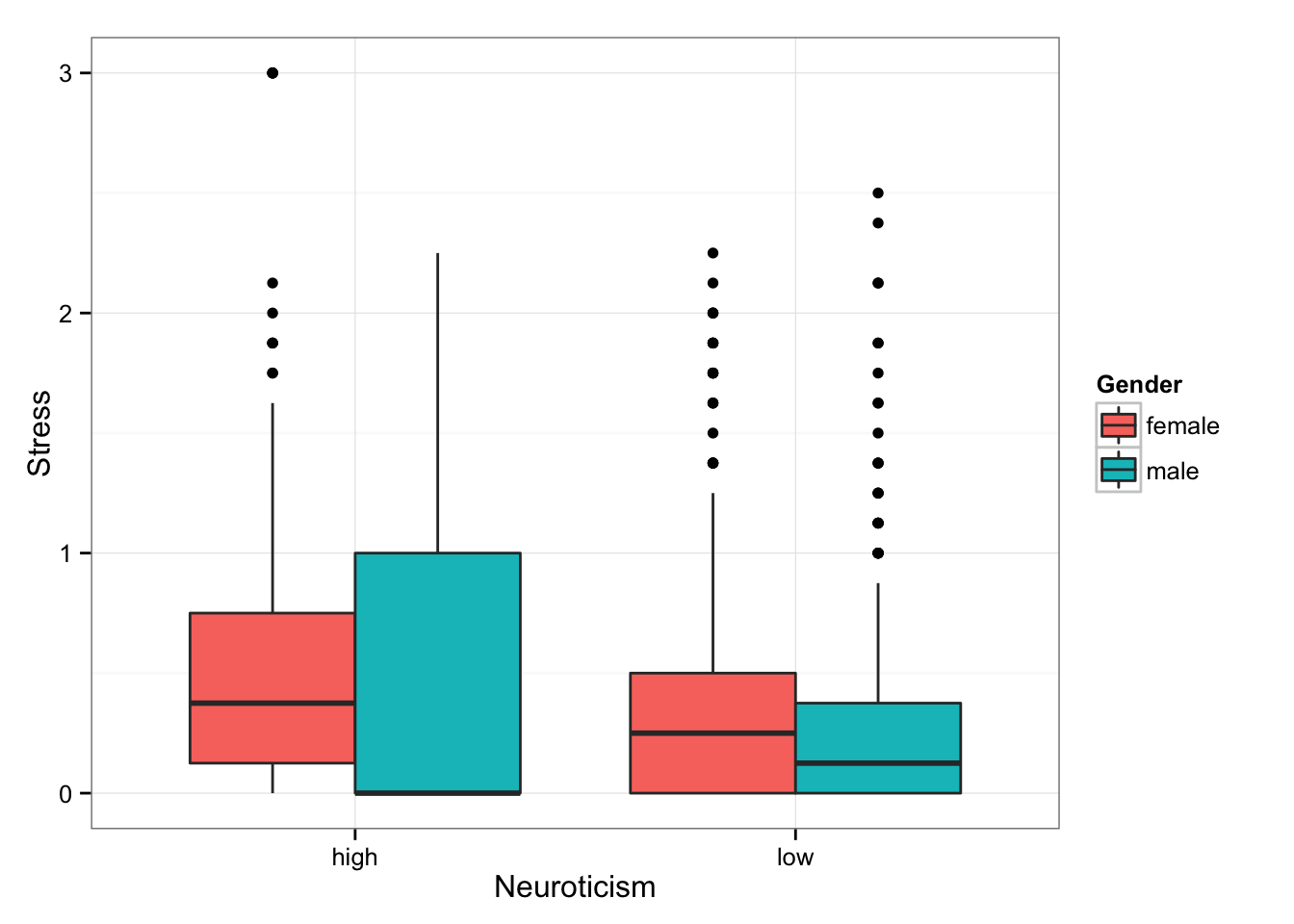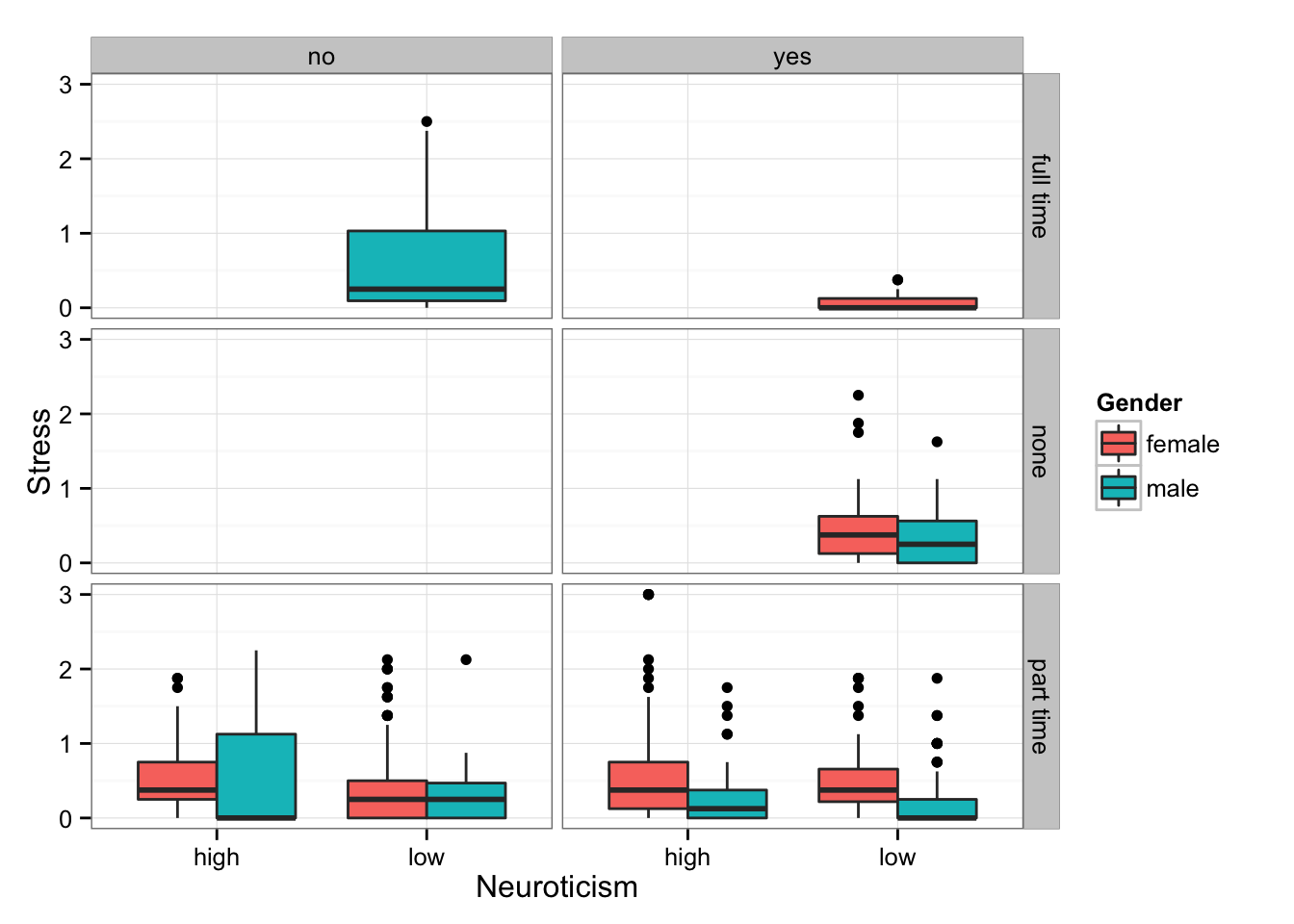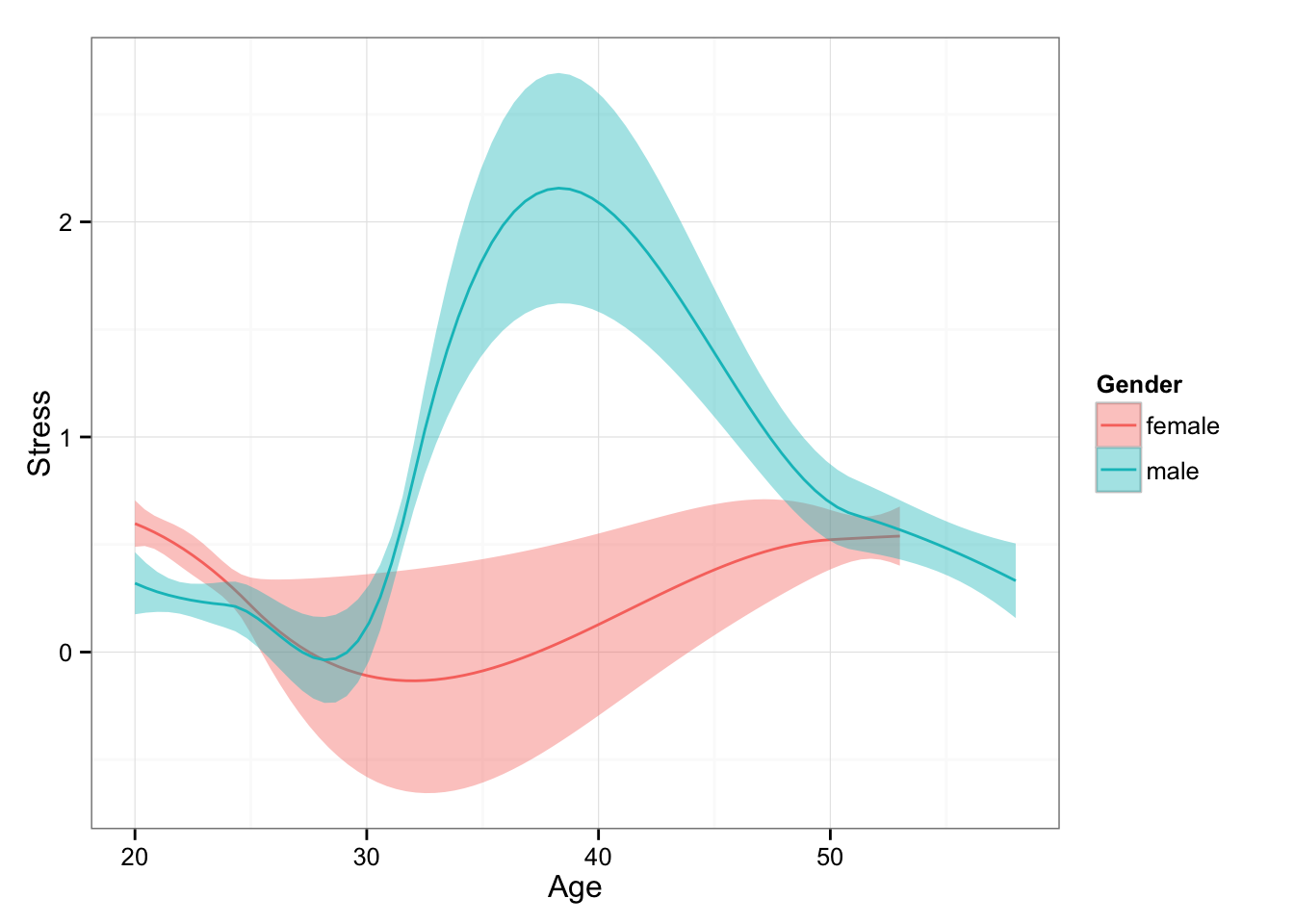The following code loads the data discussed in the lecture:

``````File <- "http://sachaepskamp.com/files/OPdata.csv"

Exercise 1: Replicate the following plot:Exercise 2: Replicate the following plot:Exercise 3: Replicate the following plot (column blocks correspond to study yes or no, row blocks to working):Exercise 4: Replicate the following plot:The following code computes the mean and standard deviation of stress levels per person:

``````library("dplyr")
DataSummarized <- Data %>% group_by(userID) %>% summarize(
mean = mean(Stress),
sd = sd(Stress),
Gender = Gender,
Neuroticism = Neuroticism
)``````

Exercise 5: Replicate the following plot: